# Maths - Homotopy Type Theory / Univalence Axiom

### Equality of Types

On the page here we looked at a type theory approach to equalities between types.

Here we look at a different approach based on the ideas of Vladimir Voevodsky and others. These ideas bring together theories from homotopy and from type theory.

 Lets take a very simple example, comparing two element sets, one set has the elements 'true' and 'false' and the other set has the elements '0' and '1'. One approach, which we might call 'extensional equality', is to say that two sets are equal if they contain exactly the same elements. In this case the elements are different so, these sets are not extensionally equal.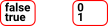A different way to define 'equality' between these sets is 'isomorphism'. They are isomorphic if we can construct arrows in each direction that allow us to get back to where we started: IdA = G•F F•G = IdB By this measure these two sets would be isomorphic. For more information see category theory pages here.We now introduce Voevodsky's 'univalence axiom' (A=B)(AB). (identity is equivalent to equivalence).

Here equivalence is like isomorphism. So the 'univalence axiom' seems like nonsense because, as defined above isomorphism and equality are different.

One way to interpret this might be that isomorphism is equality in multiple ways.

 For instance, in our example, if we set: 0 = false 1 = true then the sets are equal.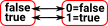Alternatively if we set: 0 = true 1 = false then the sets are equal in a different way.For more information about this 'equality' between Boolean (two element sets) and how these ideas can be extended to other types see the page here.

### Homotopy and Type Theory

The structure described above can be related to homotopy theory.

Type Theory Homotopy Theory

A type
a:A
x:AB(x)
(x:A)× B(x)
x=y

A space
a point in A
Fibration
Space of sections
Total space of fibration
Path space A

Homotopy theory is discussed on the page here.

### Type Theories

 There are various type theories including: Extensional type theory. Intensional type theory Homotopy Type Theory (HoTT) The difference between these is the relationship between equalities such as extensional equality and intentional equality.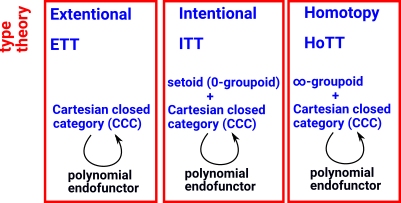### Homotopy Types

In this section we just think about how mathematical structures could be related to topology and homotopy. These models may not work for higher order paths (coherence problem) so more indirect models have been developed (see cubical types page).

So this is just for intuition.

 A set is just a discrete topology. Here is a set with 5 terms. So set theory is part of HoTT.Natural numbers (with addition) have additional structure. So in addition to the terms, represented by points, we now have lines linking things which are equal. I'm not sure this diagram fully shows the shape of natural numbers? Does it show the '1' in '1+1' is the same as the point 1.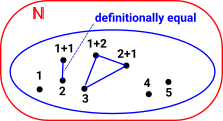Could we instead characterise the topology as open sets for the numbers (0 dimensional points) linked by 1 dimensional lines which is the successor function.## Univalence Axiom

### Properties

There is an idea that, if A and B are isomorphic, then A and B should have the same properties.

So if 'A' is some structure then ' φ(A)' is a statement in first order logic about A.

If two things are isomorphic then all properties of A are also true of B:

AB -> P(A) <=> P(B)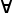P

Some properties, such as: 'contains empty set', don't obey this principle. So set theory violates this. The properties we want are invariant properties.

 In Homotopy Type Theory (HoTT) these invariant properties are homotopy invariant properties such as: path connected components number of n-dimensional holes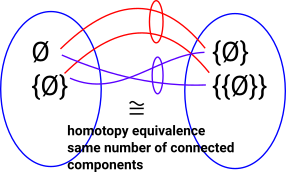Mathematicians want to find a foundation for mathematics that satisfies this principle.

This would make it easier to compute mathematics (automate proof checking and so on). Set theory axiomisation (formal set theory) is hard to work with because:

• Has properties which are not invariant.
• Requires arbitrary choices
• Axioms don't tell you how to construct structure - It would be better to have formal system in some constructive system of mathematics (to prove something we construct it).
• Set theory is built on top of logic.

Mathematicians that have worked on this problem:

• Lawvere
• McLarty
• Makkai
• Voevodski

Voevodski approach is to formalise all of maths as homotopy types (everything is a shape).

### H-Levels

H     invariant
4 2-cat   3D hole
3 category theory

equalities can be equal2D hole
2 set Can be equal in many ways path connected components
1 pointIntuitional logic
prepositional equality

Can be equal in one way

0 point

A set in homotopy is a discrete space (so set theory is contained in homotopy theory)

### Example

 Imagine we have 2 sets, A and B, which are equivalent.This induces 'equivalence classes'. So the equivalence of two sets: AB induces a type of equality of members of the set: a1 = a2 a3 = a4## Groupoid

 A non-dependant type can be represented by a groupoid.For a dependant type we need something more complicated, an ∞-groupoid.There are multiple ways things can be equal.We can start to see how the type of equality discussed on this page has the structure of groupoids by looking at the examples on the page here.

### Coding Groupoids

How could we implement these groupoids in computer code?

We need an inhabited type for each pair of terms that are equal but no type for pairs of terms that are not equal. Generating and using such a potentially large collection of types seems messy and not very workable. A family of types seems best implemented as dependant types but how can this be done for some pairs of terms but not others?

## Simplical Sets

### Combinatorial Definition

A simplical set consists of a sequence of sets X0, X1 … and, for each n≥0, functions di : Xn->Xn-1 and si : Xn->Xn+1 for each i with 0≤i≤n such that:

• didj=dj-1di if i<j
• disj=sj-1di if i<j
• djsj=dj+1sj=id
• disj=sjdi-1 if i>j+1
• sisj=sj+1si if i≤j

### Categorical Definition

Similar to combinatorial definition using order-preserving functions:

• Di : [n] ->[n+1]
• Si : [n+1] ->[n]

### Next

• For more information about this 'equality' between Boolean (two element sets) and how these ideas can be extended to other types see the page here.
• For a discussion about how this can be modeled using Cubical Type Theory see the page here.

Book Shop - Further reading.

Where I can, I have put links to Amazon for books that are relevant to the subject, click on the appropriate country flag to get more details of the book or to buy it from them.Computation and Reasoning - This book is about type theory. Although it is very technical it is aimed at computer scientists, so it has more discussion than a book aimed at pure mathematicians. It is especially useful for the coverage of dependant types.